# Kerala Syllabus 8th Standard Maths Solutions Chapter 10 Statistics

## Kerala State Syllabus 8th Standard Maths Solutions Chapter 10 Statistics

### Statistics Text Book Questions and Answers

Textbook Page No 184

Class 8 Maths Chapter Statistics Kerala Syllabus Question 1.
The number of members in 50 households of a village are listed below.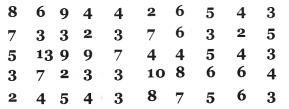Make a frequency table and answer these questions:
i. How many households have just two members?
ii. How many households have four or less?
iii. How many households have ten or more?
iv. Households of what size occurs the most?
Solution:i. 5
ii. 5 + 11 + 9 = 25
iii. 1 + 1 = 2
iv. 3

8th Standard Maths Statistics Kerala Syllabus Question 2.
There are 44 children in class 8B. The list shows how far they come from, in kilometres.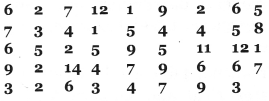Make a frequency table and answer these questions:
i. How many children are from exactly 1 kilometre away?
ii. How many are from more than 5 kilometres?
iii. How many are from between 5 and 10 kilometres?
iv. How many are from more than 10 kilometres?
Solution: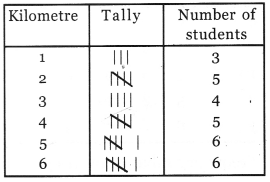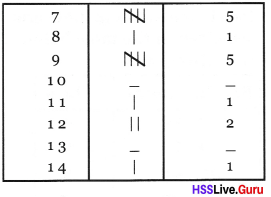i. 3
ii. 21
iii. 23
iv. 48th Standard Maths Guide Kerala Syllabus  Question 3.
The scores of 35 children in a test are given below: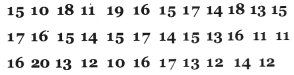Make a frequency table and answers these questions:
i. How many children scored 20?
ii. How many children got scores between 10 and 20?
iii. How many scored less than 10?
iv. What is the score most number of children got?
Solution:
Score Tally Number of children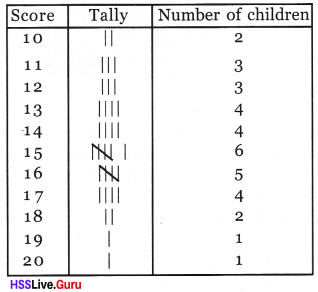i. 1
ii. 32
iii. 0
iv. 15

Textbook Page No 188

Statistics Class 8 Notes Kerala Syllabus Question 4.
Given below are the highest temperatures (in degree Celsius) one day in 40 towns. Make a frequency table.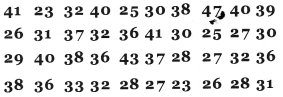Solution: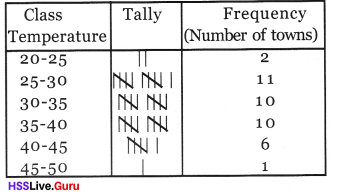8th Class Maths Statistics Kerala Syllabus Question 5.
The heights (in centimeters) of 45 people who look part in a physical fitness test are given below? Make a frequency table.Solution: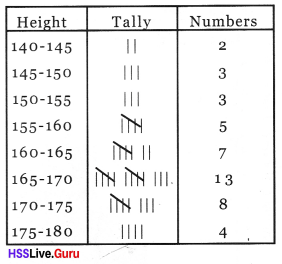Textbook Page No 190

Hss Live Guru Maths 8th Kerala Syllabus Question 6.
The table shows the times 30 children took to complete a long distance race. Draw a histogram of this.Solution: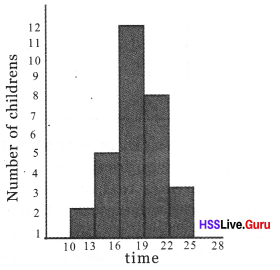Hsslive Guru Maths 8th Standard Kerala Syllabus Question 7.
The table shows the daily incomes of 6o households in a locality.Draw a histogram.
Solution: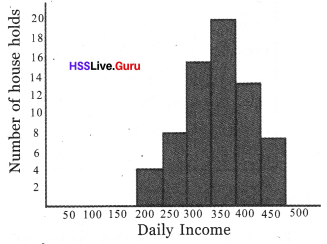Hss Live Std 8 Maths Kerala Syllabus Question 8.
Detail of rainfall in June and July are given in the table below. Draw a histogram.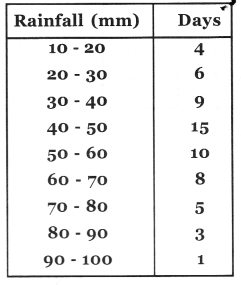Solution: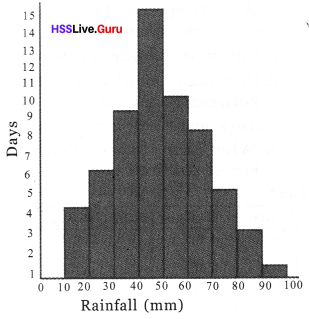Hsslive Guru 8th Class Maths Kerala Syllabus Question 9.
The time taken by 25 women and 23 men to complete a race are given in the table below. Draw separate histograms for men and women.Solution:Kerala Syllabus 8th Standard Maths Notes Question 10.
The weights of 45 children in a class are listed below.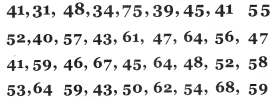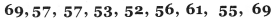Make a frequency table and draw a histogram.
Solution:8th Standard Maths Kerala Syllabus Question 1.
Complete the table below on the basis of the histogram.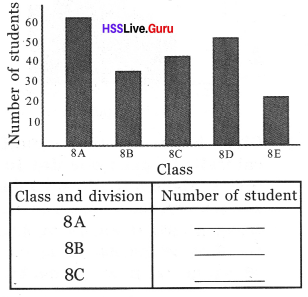Solution: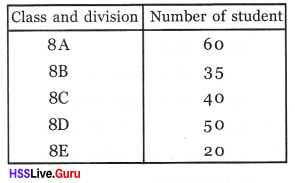8th Class Maths Notes Kerala Syllabus Question 2.
Teacher conducted a test in her class of 45 students. Their score out of a total of 10 are given below.a. Construct a frequency table representing these details?
b. Construct a bar graph?
Solution: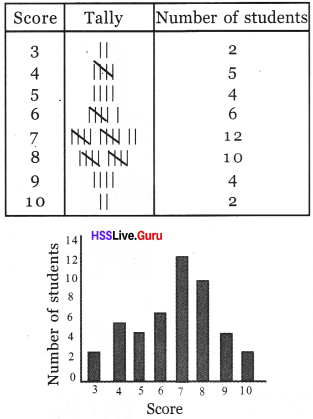Hss Live Guru Class 8 Maths Kerala Syllabus Question 3.
The list below gives the daily wages earned by 30 laboures. Prepare a frequency table of these.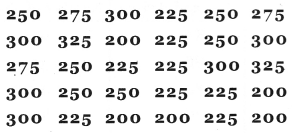a. What is the daily wages of most labourers?
b. How many get 250 rupees a day?
c. How many get the least amount of wages?
Solution: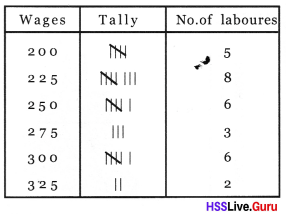a. 225
b. 6
c. 5

Class 8 Maths Chapter 10 Kerala Syllabus Question 4.
The table shows daily expenditure of 60 household in a locality. Draw histrogram.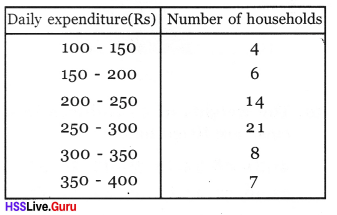Solution:8th Standard Maths Notes Kerala Syllabus Question 5.
Given below are the amount of rainfall (in mm) one day in 61 towns. Make a frequency table.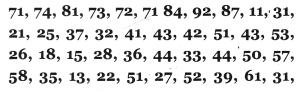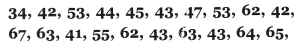Solution: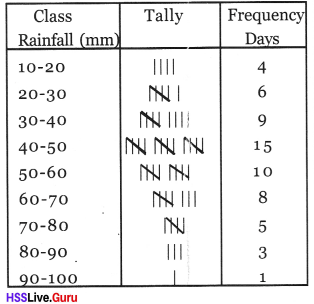8th Std Maths Solution Guide Kerala Syllabus Question 6.
The runs that a batsman got in 40 one – day cricket matches are given below. Make a frequency table and answer these questions.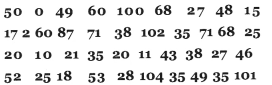a. How many centuries did he get?
b. How many half centimes?
c. In how many games did he score less than 50?
Solution: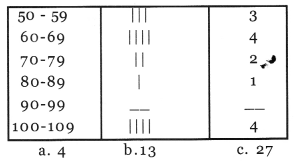Question 7.
The height of 30 childrens in a class are listed below. Mark a frequency table and draw a histogram.Solution: